## 集合 (Sets)

• ℝ 实数集合
实数包含有理数 和无理数，有理数包括整数和分数，无理数就是无限不循环小数

• ℝ+ 非负实数集合
理解了实数集，这个非负实数集合就好理解了，就是把把负数去掉

• ℝ2 有序对，像二维的坐标，就属于一个有限对，(x,y)

• ℝn 在n维笛卡尔空间中的点

• ℤ 整数集合

• S2 在单位球面上的3D点

## 映射 (Mappings)

f:ℝ↦ℤ 这个东东，可以这样解释，有一个函数叫做f，这个函数呢，接收一个输入，这个输入是ℝ，也就是上面说到的实数类型的数，注意，这里是输入一个实数，而不是输入实数集合，这里的ℝ可以理解成编程函数中的数据类型。然后呢输出一个整数类型的数。基本上可以当作编程语言中的函数调用。

## 区间 (Intervals)

• [1, 10] 表示区间范围是从 1到10
• (1, 10) 表示区间范围是从 2到9
• (1, 10] 表示区间范围是从 2到10
• [1, 10) 表示区间范围是从 1到9

并集: 给定两个集合A，B，把他们所有的元素合并在一起组成的集合，叫做集合A与集合B的 并集，记作A∪B，读作A并B。

交集: 给定两个集合A，B，把所有即属于A，又属于B的元素拿出来，组成一个新的集合，这个集合，就叫做A与B的 交集，记作A∩B。

## 二次方程 (Quadratic Equations)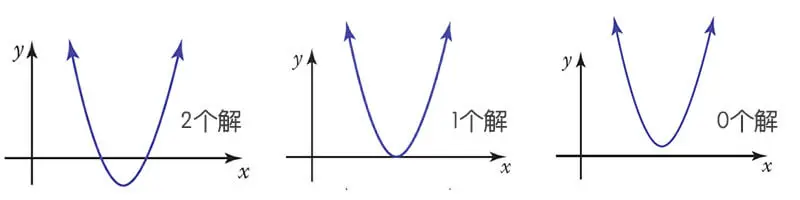## 角 (Angles)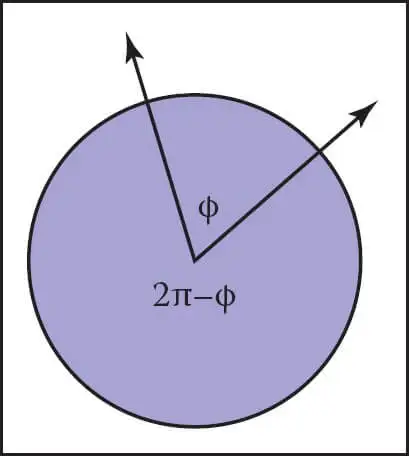## 三角函数 (Trigonometric Functions)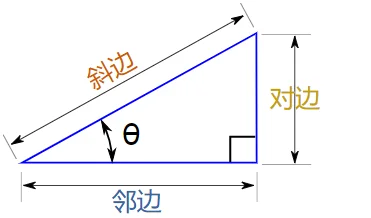1. 对边，就是对面的边，
2. 邻边，就是旁边的边，
3. 斜边，就是整个三角形最长的边。

## 向量的操作 (Vector Operation)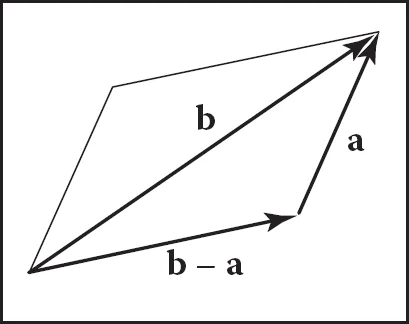负向量就是一个向量大小相同，方向相反的一个向量，例如你现在朝东，那负向量就是朝西。

## 向量的大小(模)

$\lVert x \rVert = \sqrt{x_{a}^2 + y_{a}^2}$

## 向量的点积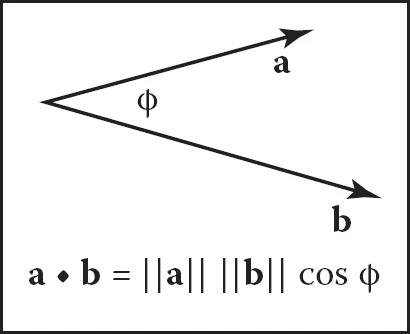$a \cdot b = \sum_{i=1}^{n}a_{i}b_{i}$

## 向量的叉积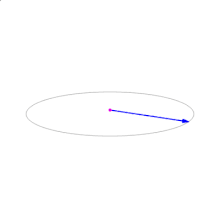叉积可以用于计算法向量。

$(x_1, y_1, z_1) \times (x_2, y_2, z_2) = (y_{1}z_{2} - z_{1}y_{2},z_{1}x_{2} - x_{1}z_{2},x_{1}y_{2} - y_{1}x_{2})$